# Basics of Inheritance CS 5010 Program Design Paradigms

• Slides: 24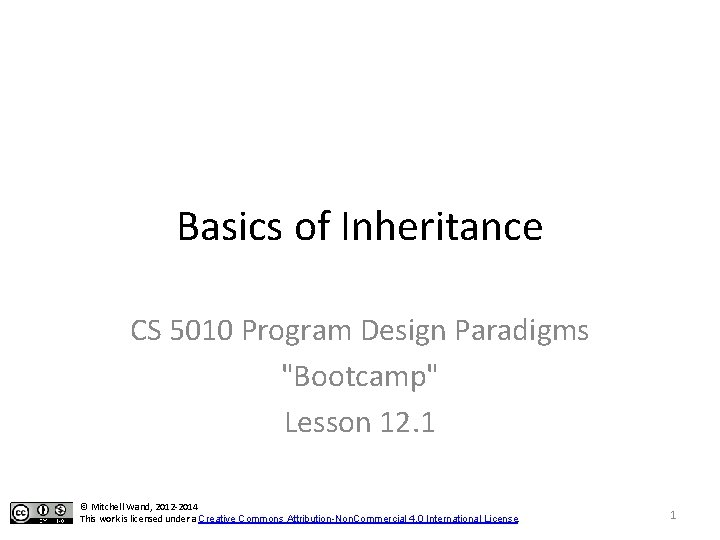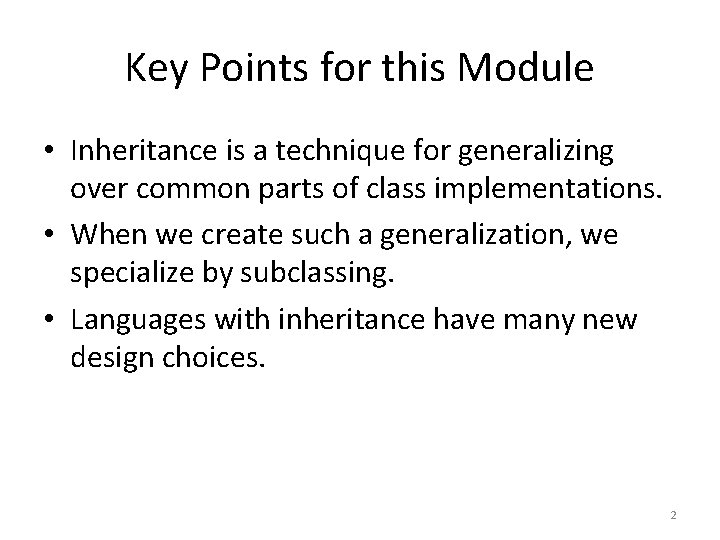Key Points for this Module • Inheritance is a technique for generalizing over common parts of class implementations. • When we create such a generalization, we specialize by subclassing. • Languages with inheritance have many new design choices. 2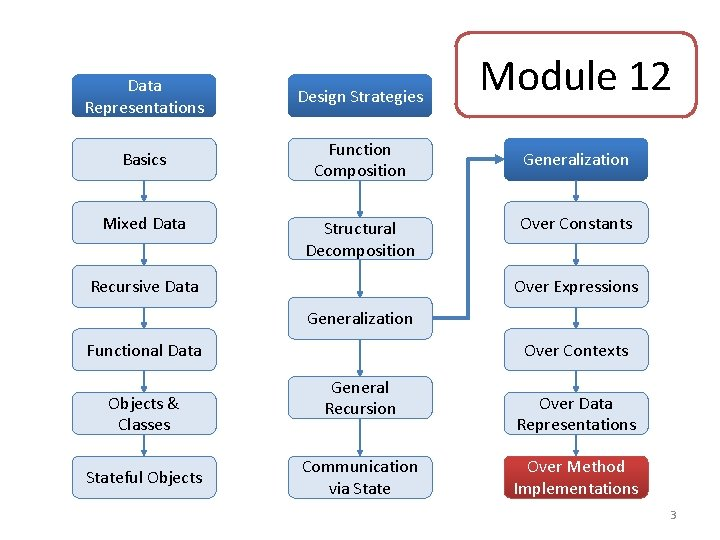Data Representations Design Strategies Basics Function Composition Mixed Data Structural Decomposition Recursive Data Module 12 Generalization Over Constants Over Expressions Generalization Functional Data Objects & Classes Stateful Objects Over Contexts General Recursion Communication via State Over Data Representations Over Method Implementations 3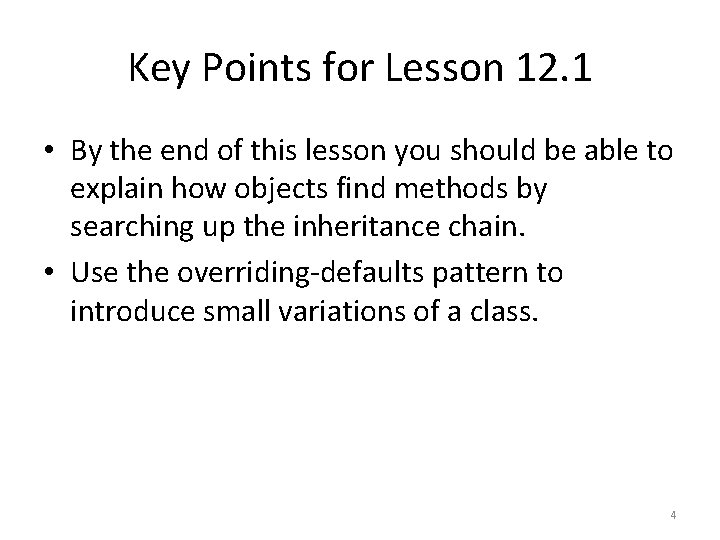Key Points for Lesson 12. 1 • By the end of this lesson you should be able to explain how objects find methods by searching up the inheritance chain. • Use the overriding-defaults pattern to introduce small variations of a class. 4Example: flashing-balls • Sometimes we want to define a new class that is just a small variation of an old class. • For example, we might want to make a ball that flashes different colors. • To do this, create a subclass that inherits from the old class (the "superclass"). • We call this the "overriding defaults" pattern. • Let's look at a demonstration. 5Video demo: flashing-balls. rkt • http: //youtu. be/YX 5 i. FECva 1 I (7: 56) 6Features for Inheritance in Racket • The Racket object system uses two features to implement inheritance: define/override and inherit-fields. – define/override is used to define methods that override methods in the superclass. – inherit-fields is used to declare fields of the superclass that we want to make visible in the subclass. • eg: x, y, selected? , radius in Flashing. Ball%. • values are automatically supplied to the superclass on initialization. Other languages do this differently, so watch out! 7What fields are in the subclass? • The init-fields of a subclass are the init-fields of the superclass plus any additional init-fields declared in the subclass. • Flashing. Ball% doesn't declare any new init-fields, so its init-fields are the same as those of Ball%. • init-fields of the subclass are automatically sent to the superclass, so when we create a Flashing. Ball%, we write (new Flashing. Ball% [x. . . ][y. . . ][box. . . ][speed. . . ]) • Those values become the values for the fields in Ball%, so they can be used by the methods in Ball%. • x and y are also inherited fields, so they are visible to the methods in Flashing. Ball% as well. 8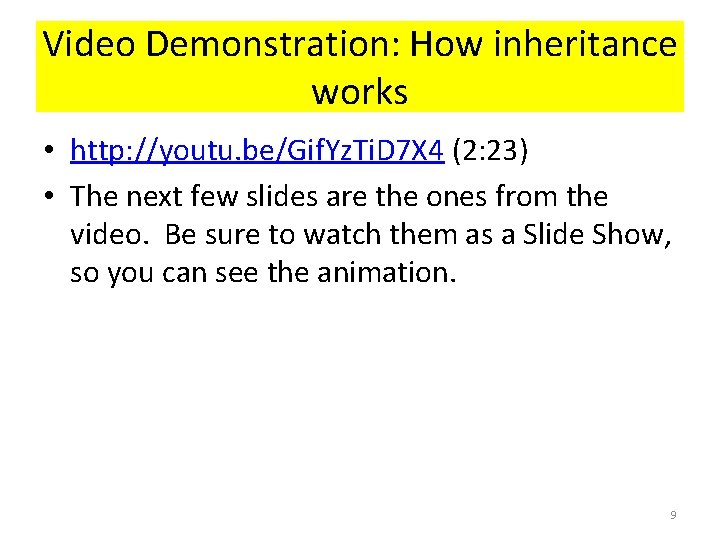Video Demonstration: How inheritance works • http: //youtu. be/Gif. Yz. Ti. D 7 X 4 (2: 23) • The next few slides are the ones from the video. Be sure to watch them as a Slide Show, so you can see the animation. 9Review: Every object knows its class (class* () (init-field x y r) (field [IMG. . . ][BOMB-SPEED. . . ]) (define/public (after-tick). . . ) (define/public (after-mouse-event. . . ) x = 10 y = 20 r = 10 x = 10 y = 20 HELI-SPEED = (class* () (init-field x y) (field [HELI-IMG. . . ][HELI-SPEED. . . ]) (define/public (after-tick). . . ) (define/public (after-mouse-event. . . ) 10Review: Every object knows its class (class* () (init-field x y r) (field [IMG. . . ][BOMB-SPEED. . . ]) (define/public (after-tick). . . ) (define/public (after-mouse-event. . . ) x = 10 y = 20 r = 10 (send after-tick) x = 10 y = 20 HELI-SPEED = (class* () (init-field x y) (field [HELI-IMG. . . ][HELI-SPEED. . . ]) (define/public (after-tick). . . ) (define/public (after-mouse-event. . . ) 11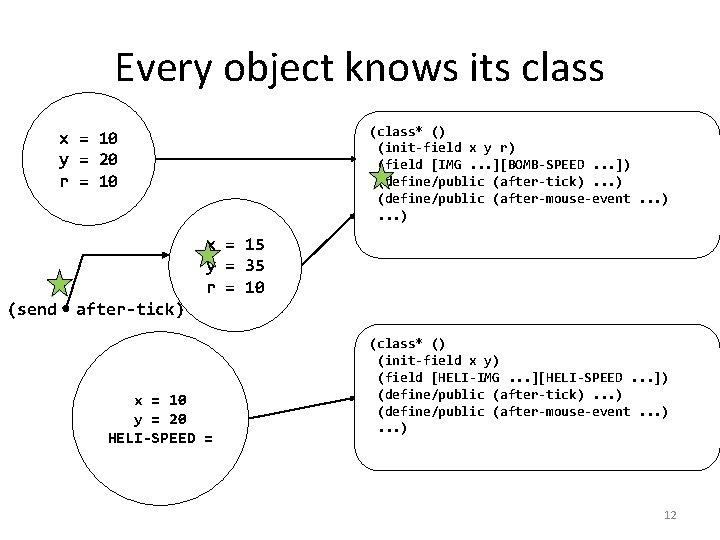Every object knows its class (class* () (init-field x y r) (field [IMG. . . ][BOMB-SPEED. . . ]) (define/public (after-tick). . . ) (define/public (after-mouse-event. . . ) x = 10 y = 20 r = 10 x = 15 y = 35 r = 10 (send after-tick) x = 10 y = 20 HELI-SPEED = (class* () (init-field x y) (field [HELI-IMG. . . ][HELI-SPEED. . . ]) (define/public (after-tick). . . ) (define/public (after-mouse-event. . . ) 12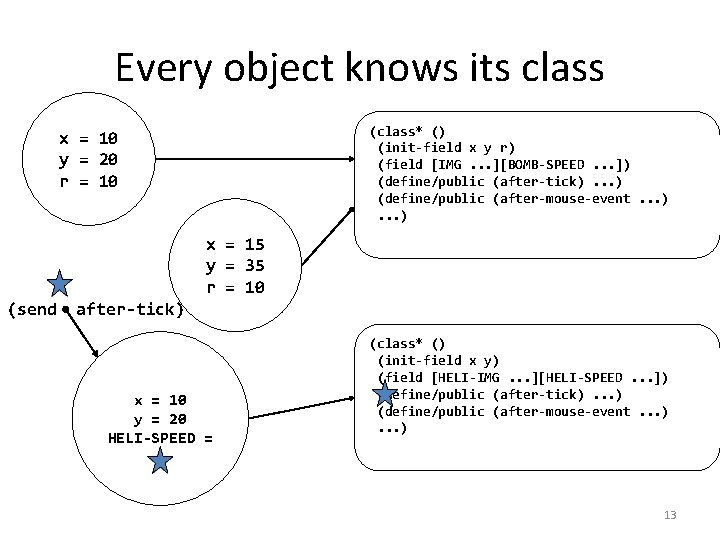Every object knows its class (class* () (init-field x y r) (field [IMG. . . ][BOMB-SPEED. . . ]) (define/public (after-tick). . . ) (define/public (after-mouse-event. . . ) x = 10 y = 20 r = 10 x = 15 y = 35 r = 10 (send after-tick) x = 10 y = 20 HELI-SPEED = (class* () (init-field x y) (field [HELI-IMG. . . ][HELI-SPEED. . . ]) (define/public (after-tick). . . ) (define/public (after-mouse-event. . . ) 13An object searches its inheritance chain for a suitable method Ball% = (class* object% () (define b 1 (new Flashing. Ball%. . . )) (send b 1 add-to-scene s) (send b 1 on-tick) (field x y radius selected? ) (define/public (on-tick). . . ) (define/public (on-mouse. . . ) (define/public (add-to-scene s). . . ) (send b 1 launch-missiles) b 1 Flashing. Ball% = (class* Ball% () (inherit-field x y radius selected? ) (field time-left. . . ) x =. . . y =. . . radius =. . . selected =. . . time-left =. . . (define/public (on-tick). . . ) (define/public (on-mouse. . . ) (define/override (add-to-scene s) (if (zero? time-left). . . ) (place-image. . . x y s)). . . ) 14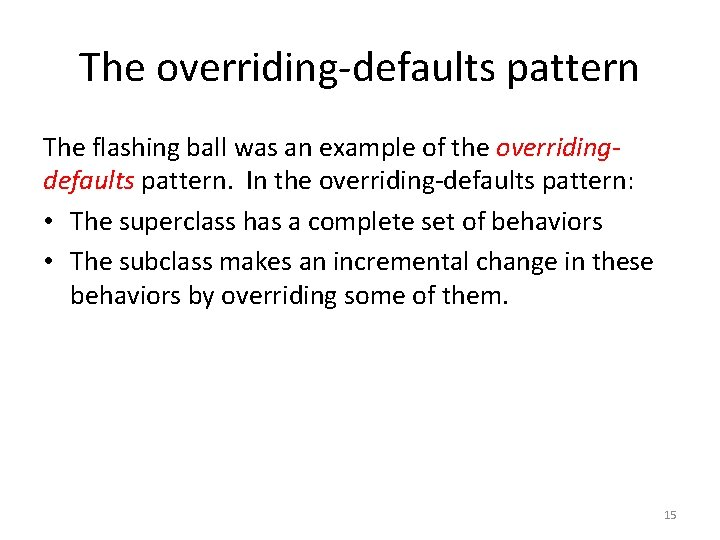The overriding-defaults pattern The flashing ball was an example of the overridingdefaults pattern. In the overriding-defaults pattern: • The superclass has a complete set of behaviors • The subclass makes an incremental change in these behaviors by overriding some of them. 15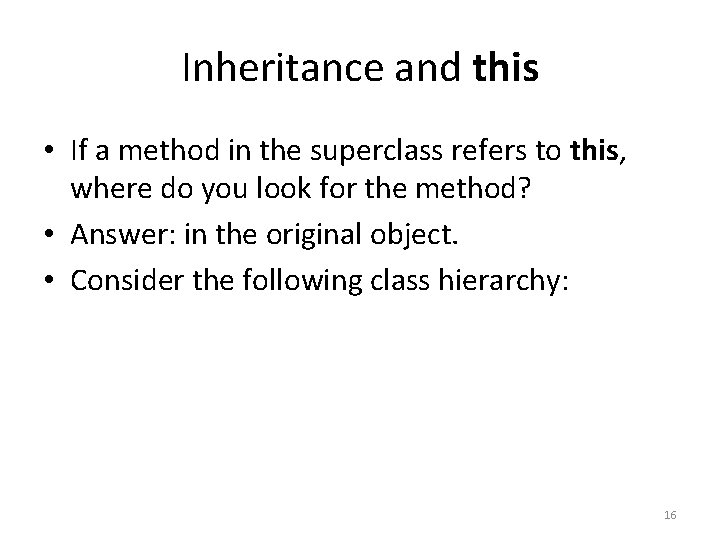Inheritance and this • If a method in the superclass refers to this, where do you look for the method? • Answer: in the original object. • Consider the following class hierarchy: 16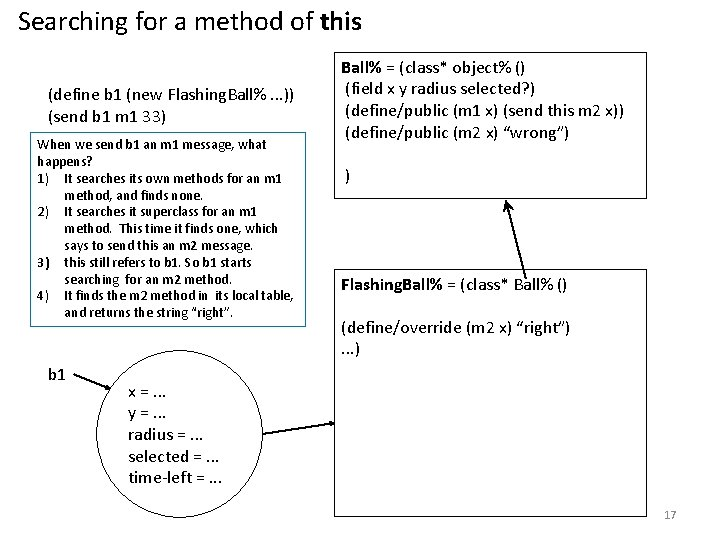Searching for a method of this (define b 1 (new Flashing. Ball%. . . )) (send b 1 m 1 33) When we send b 1 an m 1 message, what happens? 1) It searches its own methods for an m 1 method, and finds none. 2) It searches it superclass for an m 1 method. This time it finds one, which says to send this an m 2 message. 3) this still refers to b 1. So b 1 starts searching for an m 2 method. 4) It finds the m 2 method in its local table, and returns the string “right”. b 1 Ball% = (class* object% () (field x y radius selected? ) (define/public (m 1 x) (send this m 2 x)) (define/public (m 2 x) “wrong”) ) Flashing. Ball% = (class* Ball% () (define/override (m 2 x) “right”). . . ) x =. . . y =. . . radius =. . . selected =. . . time-left =. . . 17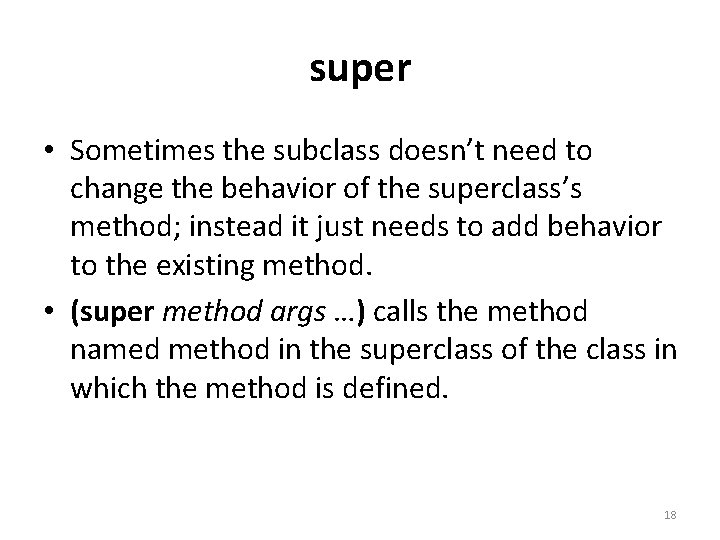super • Sometimes the subclass doesn’t need to change the behavior of the superclass’s method; instead it just needs to add behavior to the existing method. • (super method args …) calls the method named method in the superclass of the class in which the method is defined. 18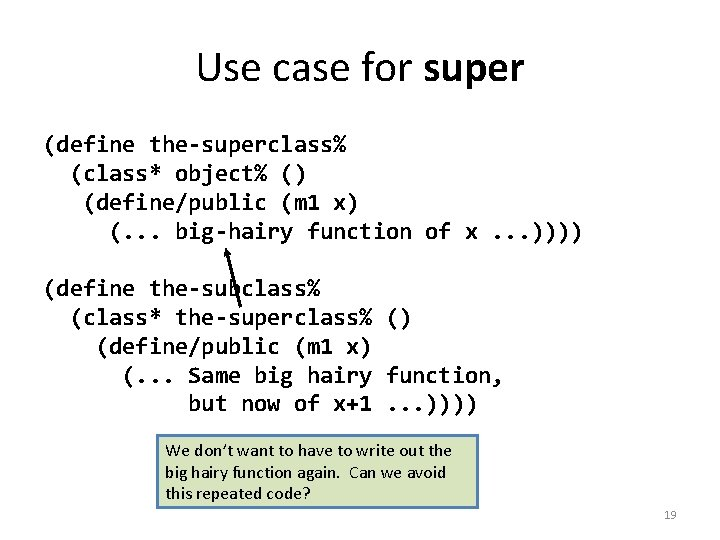Use case for super (define the-superclass% (class* object% () (define/public (m 1 x) (. . . big-hairy function of x. . . )))) (define the-subclass% (class* the-superclass% () (define/public (m 1 x) (. . . Same big hairy function, but now of x+1. . . )))) We don’t want to have to write out the big hairy function again. Can we avoid this repeated code? 19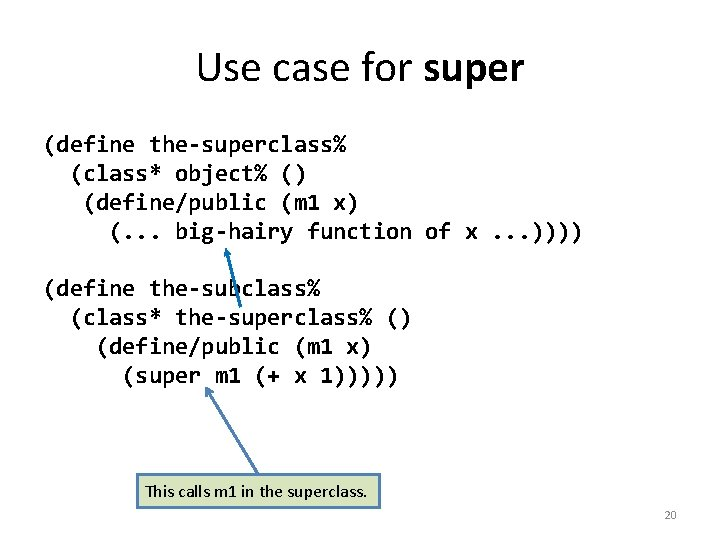Use case for super (define the-superclass% (class* object% () (define/public (m 1 x) (. . . big-hairy function of x. . . )))) (define the-subclass% (class* the-superclass% () (define/public (m 1 x) (super m 1 (+ x 1))))) This calls m 1 in the superclass. 20You can call any method in the super (define the-superclass% (class* object% () (define/public (m 1 x) (. . . big-hairy function of x. . . )))) (define the-subclass% (class* the-superclass% () (define/public (m 2 x) (super m 1 (+ x 1))))) Here method m 2 in the subclass calls method m 1 in the superclass. 21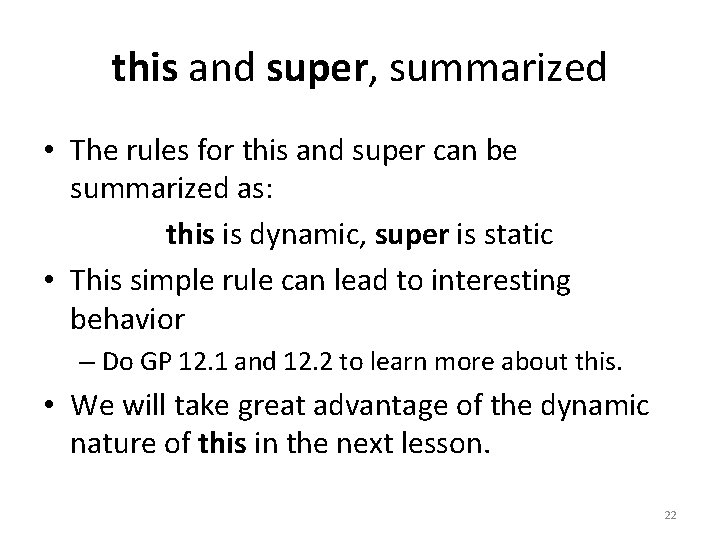this and super, summarized • The rules for this and super can be summarized as: this is dynamic, super is static • This simple rule can lead to interesting behavior – Do GP 12. 1 and 12. 2 to learn more about this. • We will take great advantage of the dynamic nature of this in the next lesson. 22Summary of Lesson 12. 1 • We’ve seen how to define superclasses and subclasses in Racket, including inherit-field and define/override. • We’ve seen the overriding-defaults pattern, in which a subclass overrides some methods of a complete superclass • We learned how this works with inheritance, and what super does. 23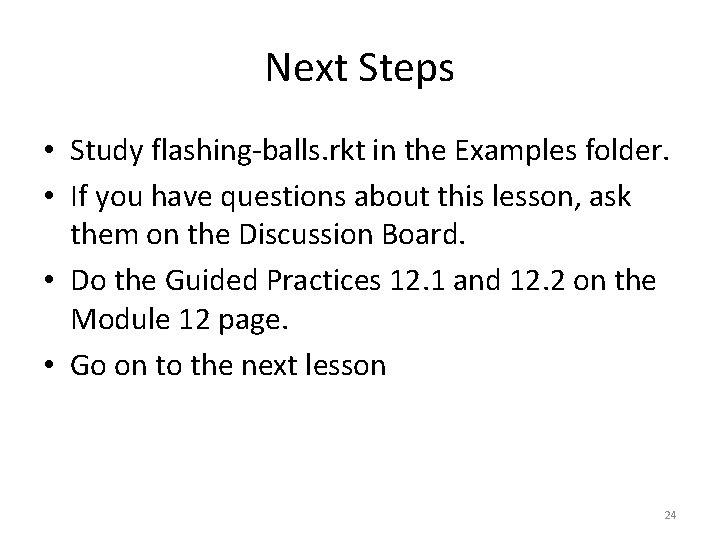Next Steps • Study flashing-balls. rkt in the Examples folder. • If you have questions about this lesson, ask them on the Discussion Board. • Do the Guided Practices 12. 1 and 12. 2 on the Module 12 page. • Go on to the next lesson 24